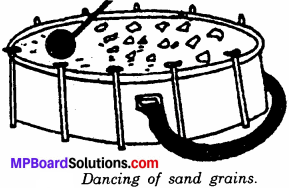## MP Board Class 6th Science Solutions Chapter 10 Motion and Measurement of Distances

### Motion and Measurement of Distances Text Book Questions

Question 1.
Give two examples each, of modes of transport used on land, water and air.

• Land: Railways, buses.
• Water: Ships, boats.
• Air: Aeroplanes, helicopters.

Question 2.
Fill in the blanks:

1. One meter is ……………… cm.
2. Five kilometer is ……………. m.
3. Motion of a child on a swing is ………………
4. Motion of the needle of a sewing machine is ………………
5. Motion of wheel of a bicycle is ……………….

1. 100
2. 5000
3. Periodic motion
4. Oscillatory motion
5. Circular motion.Question 3.
Why can a pace or a footstep not be used as a standard unit of length?
A pace or a footstep cannot be used as a standard unit of length because they are different for different people. Moreover, there will be a large difference in a pace and a footstep of child, young and an adult.

Question 4.
Arrange the following lengths in their increasing magnitude:
1 meter, 1 centimeter, 1 kilometer, 1 millimeter.
The given lengths in their increasing order are written as:
1 millimeter > 1 centimeter > 1 meter > 1 kilometer.

Question 5.
The length of a person is 1.65 m. Express it into cm and mm.
We know that,
1 m = 100 cm
and 1 m = 1000 mm
∴ 1.65 m = 1.65 × 100 cm = 165 cm
and 1.65 m = 1.65 × 1000 mm = 1650 mm.

Question 6.
The distance between Radha’s home and her school is 3250 m. Express this distance into km.
We know that,
1 m = $$\frac { 1 }{ 1000 }$$ Km
∴ Distance between Radha’s home and her school = 3250m
= 3250 × $$\frac { 1 }{ 1000 }$$ km.
= 3.250 km.Question 7.
While measuring the length of a knitting needle, the reading of the scale at one end is 3.0 cm and at the other end is 33.1 cm. What is the length of the needle?
The length of needle = 33.1 – 3.0 cm
= 30.1 cm.

Question 8.
Write the similarities and differences between the motion of a bicycle and a ceiling fan that has been switched on.

Similarity:
The motion of a bicycle and ceiling fan are in circular motion.

Dissimilarity:
The ceiling fan moves without changing its position while the bicycle moves with changing its position with time.

Question 9.
Why could you not use an elastic measuring tape to measure distance? What would be some of the problems you would meet in telling someone about a distance you measured with an elastic tape?
We should not use an elastic measuring tape to measure distance because the length of an elastic measuring tape will increase when we pull or stretch the tape for measuring the length. The measured length may be increase or decrease due to its elasticity. Thus, we cannot measure the correct length by an elastic measuring tape. The length of any object measured by this elastic tape either will be more or less depending upon the elasticity of the tape.Question 10.
Give two examples of periodic motion.
Examples of periodic motion are motion of a pendulum, a branch of a tree moving to and fro, motion of a child on a swing, strings of a guitar, etc. (any two).

Projects And Activities

Activity 1.
Work in groups and each of you do this activity one by one. Using your foot as a unit of length, measure the length and breadth of the classroom. It is possible that while measuring these you may find some part remains to be measured as it is smaller than your foot.
Measuring length and breadth of classroom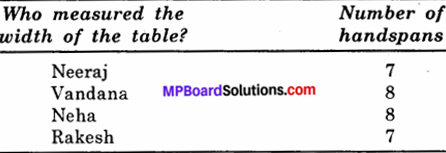Activity 2.
Work in groups and each of you use your handspan as a unit to measure the width of a table or a desk in the classroom.
Measuring width of a table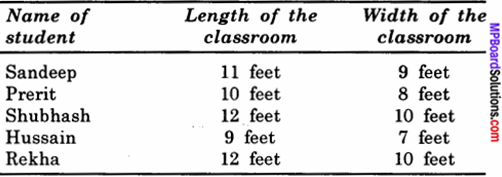Activity 3.
Think of some objects you have seen recently. List them in Table. A school bag, a mosquito, a table, people sitting on desks, people moving about? May be a butterfly, dog, cow, your hand, a small baby, a fish in water, a house, a factory, a stone, a horse, a ball, a bat, a moving train, a sewing machine, a wall clock, hands of a clock? Make your list as large as you can. Which of these are moving? Which are at rest?
Table: Objects in rest and motion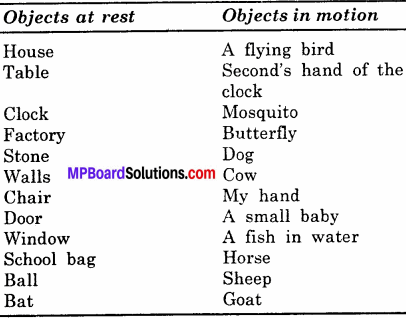### Motion and Measurement of Distances Additional Important Questions

Motion and Measurement of Distances Objective Type Questions

Question 1.

Question (i)
The SI unit of the length is –
(a) Centimeter
(b) Meter
(c) Decimeter
(d) Kilometer
(a) Centimeter

Question (ii)
The SI unit of mass is –
(a) Milligram
(b) Gram
(c) Kilogram
(d) Quintal
(c) KilogramQuestion (iii)
SI unit of time is –
(a) Day
(b) Hour
(c) Minute
(d) Second
(d) Second

Question (iv)
To read the scale the eye should be vertically –
(a) Upward
(b) Downward
(c) Both
(d) None
(a) Upward

Question (v)
Flying kite is an example of –
(a) Circular motion
(b) Periodic motion
(c) Irregular motion
(d) Linear motion
(c) Irregular motion

Question 2.
Fill in the blanks:

1. One decameter equals ……………. meters.
2. One decimeter equals ……………. centimeters.
3. One quintal equals ……………. kilograms.
4. One millennium equals ……………. centuries.
5. One metric ton equals …………… quintals.
6. Meter is indicated by sign …………….
7. Kilogram is indicated by sign …………….
8. Second is indicated by sign …………….
9. Changing of day and night is ……………. change.

1. 0.1
2. 10
3. 100
4. 10
5. 10
6. m
7. kg
8. s
9. PeriodicQuestion 3.
State whether the following statements are true (T) or false (F):

1. In ancient India, small length measurements used were an angul (finger) or a mutthi (fist).
2. In 1790, the French created a standard unit of measurement called the metric system.
3. For measuring large distances, meter is not a convenient unit.
4. Handspan is a standard unit of length.
5. Length of a curved line cannot be measured by a meter scale directly.
6. Motion of the balance wheel of a watch is a periodic motion.
7. The motion of a ball rolling on ground is random motion.
8. The motion of a point marked on the black of an electric fan is the example of circular motion.
9. The motion of the moon round the earth is periodic.
10. When a drum is struck gently, its membrane shows random motion.

1. True
2. True
3. True
4. False
5. True
6. True
7. True
8. True
9. False
10. False.

Question 4.
Match the items in Column A with Column B: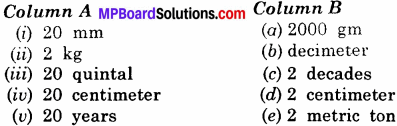(i) (d)
(ii) (a)
(iii) (e)
(iv) (b)
(v) (c).

Question 5.
Match the items in Column A with Column B: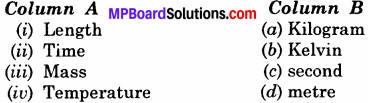(i) (d)
(ii) (c)
(iii) (a)
(iv) (b).

Motion and Measurement of Distances Very Short Answer Type Questions

Question 1.
How may kilogram are there in one metric ton?
1 metric ton = 1000 kg = 103 kg.

Question 2.
How many seconds are there in one day?
Number of seconds in one day=24 × 60 x 60 = 86400 s.

Question 3.
Why a pace or a foot step cannot be used as a standard unit of length.
The pace or a foot step vary from person to person. These methods are not satisfactory.

Question 4.
Arrange the following lengths in their increasing order: metre, centimeter, kilometer, millimeter.
Millimeter < centimeter < metre < kilometer.Question 5.
Arrange the following masses in their increasing order: kilogram, quintal, milligram, gram.
Milligram < gram < kilogram < quintal.

Question 6.
How many liters would be there in 1000 ml?
1 litre, because liter = 1000 ml.

Question 7.
How many seconds are there in 8 minutes?
∵ 1 minutes = 60 seconds
∴ 8 minutes = 8 × 60 seconds
= 480 seconds.

Question 8.
How many centimeters means 8 meters?
∵ 1 metre = 100 centimeter
∴ 8 meters = 8 × 100 centimeters
= 800 centimeters.

Question 9.
How many meters means 3000 mm?
∵ 1 mm = 100 metre
3000
∴ 3000 mm = metre = 3 metre.

Question 10.
To measure the length of chest, what instrument would you use? Can you measure chest with the help of any other method?
For measuring the size of chest we use a measuring tape. A metre scale cannot be used. It will not give correct measurement because it is not flexible.Question 11.
Why hand is not a standard unit to measure length?
A hand cannot be used as standard unit of length because the length of hand of all the people are different. So the length measured by one person will differ from the length measured by the other person.

Question 12.
What happens if the tailor takes your measurement with hand?
There is variation in the measurement takes by hand because the length of hand-span of every tailor was different.

Question 13.
State any two points necessary for measurement.
The measurement is the comparison of any two unknown quantity with same kind of definite known quantity. The measure should be such that it must have same meaning for each individual. To bring uniformity in measurement we use standard units.

Question 14.
At which place, the standard units have been preserved?
International standard units have been preserved at the Weight and Measures Bureau in Paris.

Question 15.
Where does the copies of standard units have been preserved in India?
In India, one copy of International standard units is preserved at National Physical Laboratory, Pusa Road, New Delhi.

Question 16.
Name the quantity which a shopkeeper measures while selling rice.
Kilogram.

Question 17.
Name the two parts which must be mentioned to state the results of a measurement.
The two parts are

1. Quantity
2. Unit.Question 18.
Name the SI units of length?
SI unit of length is 1 metre or 9 m.

Question 19.
Name the SI unit of mass?
SI unit of mass is kilogram or 9 kg.

Question 20.
What is motion?
Motion is the continuous change in position of a body with respect to time as compared to a stationary object. For examples, moving car, moving fan, etc.

Question 21.
Is your classroom at rest or in motion?
It is at rest because’ it is not changing its position.

Question 22.
Is the hour’s hand of a wall clock at rest or in motion?
The hour’s hand of a wall clock is moving though it moves very slowly.

Question 23.
What type of motion do the vehicles on a straight road perform?
The vehicles perform rectilinear motion on the road.

Question 24.
What type of motion does the wheel of a bicycle perform?
The wheel of a bicycle perform rotatory or circular motion.

Question 25.
An object is hung from a spring is pulled down and left. What type of motion does the object perform?
The object perform oscillatory motion which is also a periodic motion.

Question 26.
Give two examples of periodic motion.

1. Rotation of earth on its axis.
2. Moon moves around the earth.

Question 27.
Give two examples of non – uniform motion.

1. Motion of the train when its driver applies breaks.
2. Motion of the cricket ball when the bowler throws the ball and the ball is hit by the player.Question 28.
Name the three types of motion.
Three types of motion are rectilinear motion, rotatory motion and oscillatory motion.

Question 29.
Which type of motion does the feet of a tailor perform while sewing some clothes on a sewing machine?
The feet of the tailor perform periodic motion while sewing clothes on a sewing machine.

Motion and Measurement of Distances Short Answer Type Questions

Question 1.
What do you mean by measurement, explain with example?
The comparision of an unknown quantity with a known standard quantity (called unit) is known as measurement. For example, while buying things, we ask a certain amount of quantity to the shopkeeper, he then weight the exact quantity and gives it to us, like one kg sugar, 5 metre cloth, 10 kg. flour etc.

Question 2.
In our daily life, where we need measurement. Give any five examples.
Examples of measurements:

1. To measure the length of cloth before serving.
2. To measure the weight of vegetables before buying.
3. To build house.
4. To reach school in time.

Question 3.
What are the difficulties in using non – standard units?
People use foot, arm, stretched palm, etc. as units of length. These non – standard units (or methods) were not satisfactory because lengths of arm, foot and stretched palm vary from person to person. However if there are different units of length in the different parts of the world, it is very inconvenient for the exchange of scientific information. Thus the need for the standard unit been felt. The unit which people agree to use as a basic unit for measurement becomes a standard unit for the group of people for the sake of uniformity.Question 4.
What do you understand by International System of measurements? Write a short note on it.
For bringing the uniformity in units, scientists have done lot of efforts on International level and prepared an unanimous standard system. It is known as International Standard System. It is abbreviated as SI units.In this system length is measured in metre mass in kilogram and time in second. A metre is a distance equal to one million (10-7) time of the distance between equator and pole. A second is 1/86400 times of a mean solar day. A kilogram is equal to one thousand milligrams.

Question 5.
Why standard units are necessary?
A quantity adopted as a standard of measurement of physical quantity, is called a unit. In fact, the unit of measurement should be such that the magnitude measurement must be the same even if it is measured by different persons. The measure should be such that it must have same meaning for each individual. To bring uniformity in measurement standard units are necessary.

Question 6.
How can you find out that a given meter scale is correct or not?

1. A correct metre sacle has (←, →) signs on its both ends as shown in figure.
2. A correct metre scale has the stamp of the Weights and Measure Department.
3. A correct metre scale is straight, not bent from the centre.
4. A correct metre sacle gives the correct measurement of length.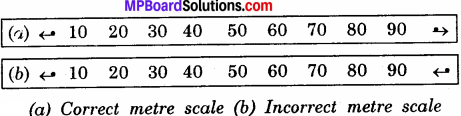Question 7.
Give three examples of rectilinear motion. Explain.

1. A scooterist driving on a road.
2. Soldiers marching in the battlefield.
3. A ball falling from a height.

In all these examples objects change their position with time along a straight line.

Question 8.
Give two examples of rotatory motion. Explain.

1. Motion of blades of a fan.
2. Motion of minute hand of the clock.
3. Motion of the wheel of a car.

In these examples the object or any parts of it move in a circular path. This is called rotatory or circular motion.

Question 9.
What are the differing types of motion?
The different types of motions are :

1. Linear motion
2. Circular motion
3. Curvilinear motion
4. Oscillatory motion
5. Random motion
6. Periodic motion

A motion can be the combination of all these types of motions or a few of them.

Question 10.
What are periodic and non-periodic motions? Explain with example.
Periodic motion:
The motion of a body which is repeated at regular intervals of time is called periodic motion. In periodic motion, a body covers equal distances in equal intervals of time. For exampls, revolution of earth round the sun, the motion of moon round the earth, the motion of a swing, the motion of a pendulum, etc.

Non – periodic motion:
The motion of a body which is not repeated at regular intervals of time is called non-periodic motion. In non – periodic motion, a body does not cover equal distances in equal intervals of time. For examples an athlete running on a track, a moving car on busy road, etc.Question 11.
How is circular motion different from curvilinear motion?
Circular motion is curvilinear motion but it involves the motion of a body at a fixed distance from a fixed point called axis. The figure explains the difference. As motion of the body ‘A’ is around the axis but in second case ‘B’ it is curvilinear motion.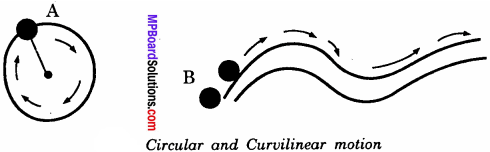Question 12.
Give a table to show multiples and sub – multiples of units of length, mass and time.
Length
10 mm = 1 centimeter (cm)
10 cm = 1 decimeter (dm)
10 decimeter = 100 cm = 1 metre (m)
10 metre = 1 decameter
100 metre = 1 hectometer
1000 metre = 1 kilometer (km)

Mass
1000 (mg) = 1 gram (g)
1000 gm = 1 kilogram (kg)
100 kg = 1 quintal
10 quintal = 1 metric ton

Time
60 seconds = 1 minute
60 minutes = 1 hours
24 hrs. = 1 day
365 days = 1 year

Motion and Measurement of Distances Long Answer Type Questions

Question 1.
What precautions are taken while measuring length?
The precautions which should be taken while using a metre scale to measure length are the following:

1. The scale should be placed along the length to be measured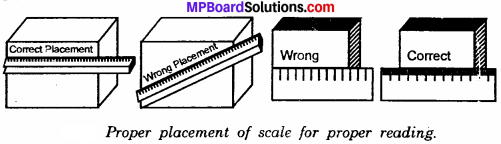2. The scale should be placed very close to the object to be measured.

3. The broken scale should not be used because if used the 1 always begin with the final mark on scale. Subtract the final reading from initial reading to get length e.g., if the initial reading is 4.2 cm and final reading is 6.8 cm. then the length is 6.8 – 4.2 cm = 2.6 cm.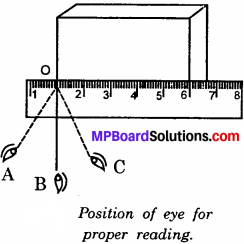4. To read to scale correctly the eye should be vertically upward. As shown in fig. OA and OC give wrong reading of length while OB gives the correct reading of length.

Question 2.
Identify the measuring instruments given in the pictures and tell where they are used?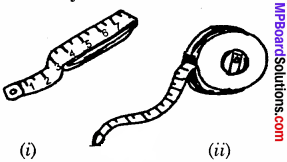1. Inch tape:
It is used for measuring height and tailor also uses this inch tape.

2. Steel tape or measuring tape:
It is used for measurement of glass, building height and carpenter also uses this.

Question 3.
Identify the correct method of keeping scale for measuring the length?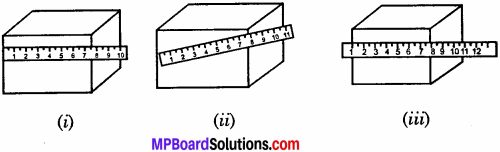1. Is the correct method of keeping scale for measuring the length.

Question 4.
With the help of thread and scale find out the length of Rs. 5 coin.
Mark a dot on one end of the circumference of the coin. Now put a knot on one end of the thread. Keep it tight on the mark on the coin. Move the thread around the circumference till the mark on the coin is reached again. Mark this position on the thread with a dark pencil. Measure the length of the thread between the knot and the mark. (Do not stretch the thread with a force). This is the length of circumference of five rupee coin.

Verify this length by keeping the thread in stretched position on the table between two tight nails. Mark a dot with a pencil. Place the rupee coin in vertical position. Now slide the coin along the length of thread till the mark on the coin is reached again as shown in the diagram below. Measure the distance between two marks on the thread. This is the circumference of the coin. The two measurement must be exactly identical.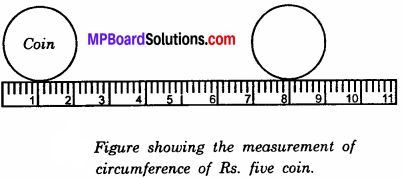Question 5.
Use a thread to measure the length of a curved line.
Mark the ends of curved line by putting full stop. Tie a knot on the open end of the thread. Place the knot of the thread on end A of the line. Now place the thread along the line keeping it tightly gripped with the help of both the thumbs or fore-fingers as in the diagram.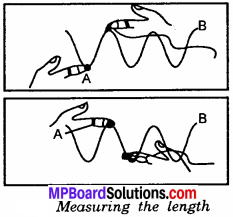Now move the thumb a little further keeping the thread in position and then press and release the hold on the thread as you keep on sliding the thread along the curves on the line. When the thread reaches the end B mark this position on the thread. You may tie the second knot at this point. Now measure the length of thread between two knots with the help of the metre scale. This is the length of the curved line.Question 6.
How many kinds of motions are? On the basis of daily experience explain only two of them.
The different types of motions are:

1. Linear motion
2. Curvi linear motion
3. Random motion
4. Circular motion
5. Oscillatory motion
6. Periodic motion
7. Non – periodic motion.

A motion can be the combination of all these types of motions or a few of them.

1. Linear motion:
It is also called as translatory motion. It involves the motion of a body along a straight line. For examples, a bullet fired from a rifle, a ball rolling on ground, a child sliding down a slope as shown in fig.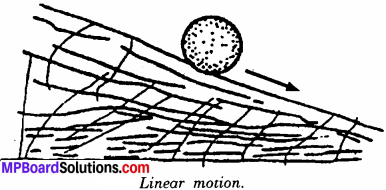2. Curvilinear motion:
The motion of a body along a curved path is called curvilinear motion. For examples, a cyclist turning on road, a ball falling from a height, a train moving on a curved rail track, football kicked high in air by a player, etc.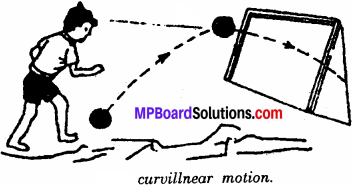3. Random motion:
The motion of a body in which it changes its position rapidly with time in all the possible directions is called random motion. For examples, the motion of a mosquito, the motion of a hockey ball or of football in the ground, etc.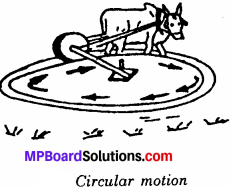4. Circular motion:
The motion of a body along a circular path about a fixed axis is called circular motion. For examples, revolution of moon around the earth, revolution of earth around the sun, a rotating spinning top, moving wheel, a moving fan, etc.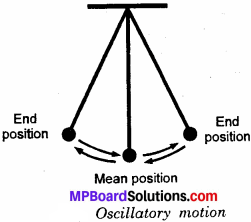5. Oscillatory motion:
A body is said to be in oscillatory motion when it moves to an fro about a fixed point. For examples, the pendulum of a wall, clock (Fig.), a swing, a wire of sitar when plucked, striking of drum, etc. Mostly the hanging objects show oscillatory motion.

6. Periodic motion:
The motion of a body which is repeated at regular intervals of time is called periodic motion. In periodic motion, a body covers equal distances in equal intervals of time. For examples revolution of earth round the sun, the motion of moon round the earth, the motion of a swing, the motion of a pendulum, etc.

7. Non – periodic motion:
The motion of a body which is not repeated at regular intervals of time is called non – periodic motion. In non – periodic motion, a body does not cover equal distances in equal intervals of time. For examples an athelete running on a track, a moving car on the busy road, etc.Question 7.
Give an experiment to show that motion of plucked string is oscillatory motion?